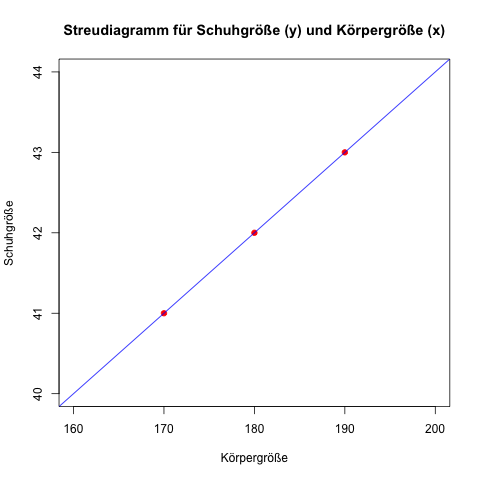# What is the intercept and slope in the regression

## Linear regression

### Example linear regression

For 3 men (Anton, Bernd and Claus) the height in cm and the shoe size are recorded. Is there a linear relationship? - and how can this be expressed in a formula? In this case, the shoe size (variable y) from the height (variable x) can be derived or forecast.

First, the arithmetic mean values ​​(averages) for body height and shoe size are calculated:

• Height: (170 cm + 180 cm + 190 cm) / 3 = 180 cm.
• Shoe size: (41 + 42 + 43) / 3 = 126/3 = 42.

Then (in the columns of the table)

• the respective deviations from the mean value are calculated for the body size
• the height deviation is squared
• the respective deviations from the mean value are calculated for the shoe size and
• the deviations from the mean are multiplied in each case.

#### Calculate the slope

Now the sum of the multiplied deviations is divided by the sum of the squared deviations in body height: 20/200 = 0.1.

As formula: β = ∑ [(xi - ∅x) × (yi - ∅y)] / ∑ (xi - ∅x)2

The slope of the regression line determined in this way corresponds to the quotient of the covariance (20/3) and the variance of the body size (200/3).

#### Calculate the intercept

As a final step, the value just calculated times the average height is subtracted from the average of the shoe size: 42 - 0.10 × 180 = 24.

As formula: α = ∅y - β × ∅x

#### Regression line

The regression line as a linear function is then: 24 + 0.1 × height.

Generally as formula:

yi = α + β × xi

Here, α (24) is the point of intersection with the y-axis (the shoe sizes in the example therefore start at 24, with the theoretical body size 0), β (0.1) is the slope of the regression line and xi or yi are the respective body and shoe sizes.

Here, α and β are also used as Regression coefficients denotes or α as Regression constant; ß indicates by how many units y increases when x increases by one unit (in the example: if the body height increases by 1 cm, the shoe size increases by 0.1 shoe sizes).

For Anton: 24 + 0.1 × 170 = 41.

For Bernd: 24 + 0.1 × 180 = 42.

For Claus: 24 + 0.1 × 190 = 43.

The regression formula can now be used to estimate or predict the shoe size (for other men) based on a body size.

The scatter diagram for the 3 measurement data including the regression line: